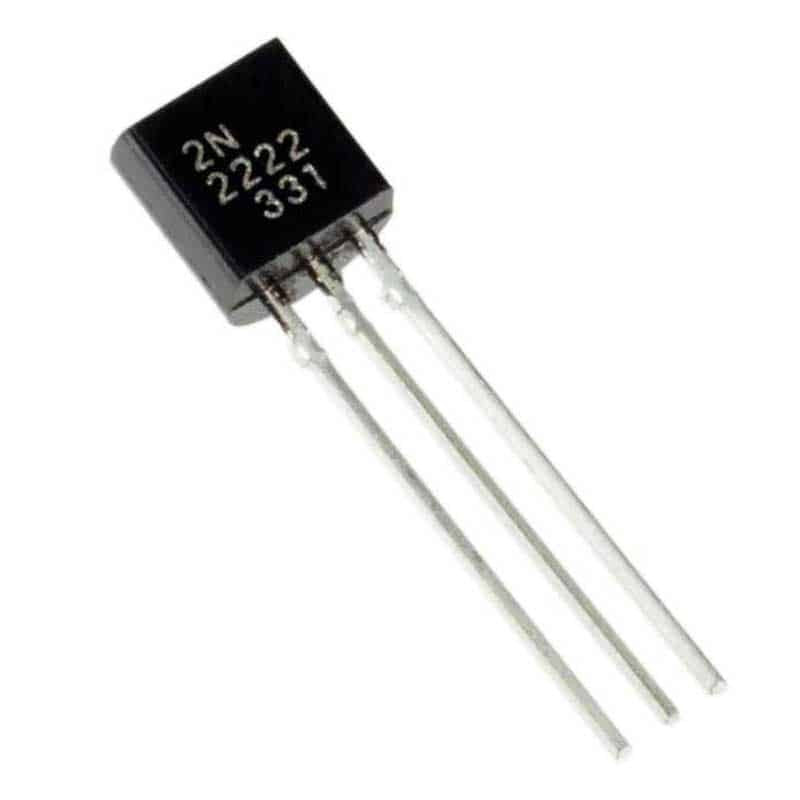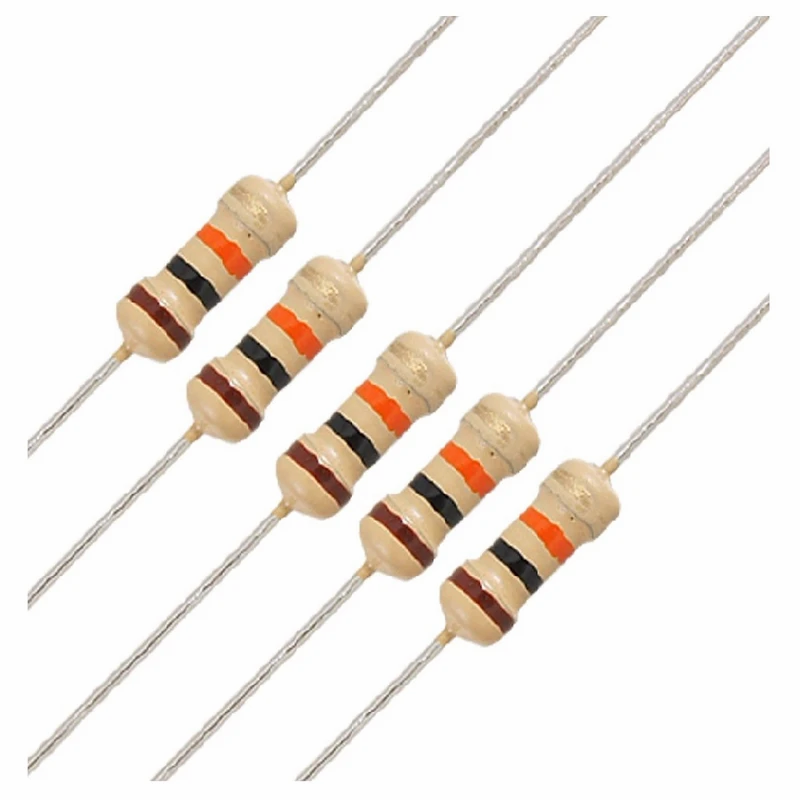• nithya7rns

# OR Gate using Transistor

Updated: Apr 8

Digital systems were developed to analyze the input and output systematically. The digital systems as the name suggests will have only two possible inputs and outputs, 0 and 1. The logic behind the system becomes easy and various circuits can be designed accordingly.

OR gate is one of the basic gates that can be used to design other logic gates. Transistor, on the other hand, is an electronic device usually used as an #amplifier or an electronic switch. Due to one of the main functions of the transistor as a switching element, they are used to design logic gates.

### What is an OR gate?

The basic working rule of OR Gate is that the output is HIGH when at least one of the inputs is HIGH. The output equals 0 when both the inputs are 0. OR Gate can also be called #Inclusive and the statement apt for describing the function of OR gate is "If either A or B is true, the output is true." The HIGH value is 1 and the LOW value is 0. The symbol used for the OR function is '+' and the Boolean expression used to denote the function is 'A + B'.

The OR gate gives the following output as shown in the truth table.

INPUT A INPUT B OUTPUT(Y)

0 0 0

0 1 1

1 0 1

1 1 1OR - Logic gate

OR Gate can be configured using other Logic gates. Here are a few examples.

Using NAND Gates.OR Gate using NAND Gates

As we know, NAND and NOR are #universal gates. It means that the combination of these gates together can form another Logic Gate. NAND gate is nothing but the negation of AND gate, that is when the output of an AND gate passes through a NOT gate, NAND gate output can be derived. The expression is given by,

For the first NAND Gate,

Input: A, Output: NAND(A) or A'

For the second NAND Gate,

Input B, Output: NAND(B) or B'

The outputs of the first two NAND Gates will be the Inputs to the third NAND Gate.

Input A', B' Output: NAND (A', B')

= (A' . B')' [NAND applied to whole expression]

= A ' ' + B ' ' [De-Morgan's Law]

= A + B [OR Gate Expression]

The truth table of the NAND gate is given below.

Input A Input B Output

0 0 1

0 1 1

1 0 1

1 1 0

The inputs to the two NAND gates get processed according to the truth table and when the output from these two NAND goes to the final NAND gate, due to a combination of these gates, the output matches that of the OR gate.

Using NOR Gates.OR Gate using NOR Gates

NOR gate is another Universal gate, the combination of which can be used to design an OR gate. The NOR gate is the negation (NOT) of the OR gate. When the outputs of the OR gate is passed through the NOT, NOR gate outputs is derived. The expression is given by,

For the first NOR Gate,

Inputs A, B. Output: (A + B)'

= A' . B'

R = A' . B'

For the second NOR Gate,

Input (A . B). Output: (A' . B')'

= A' ' + B' ' [DeMorgan's Law]

X = A + B [OR Gate expression]

The truth table for the NOR gate is given below.

Input A Input B Output

0 0 1

0 1 0

1 0 0

1 1 0

The given input first passes through a NOR gate and the output of which passes through another NOR gate which gives the output matching the OR gate.

Using a Combination of Logic Gates.OR Gate using a combination of Logic Gates.

A combination of Logic gates other than the Universal gates can also be made to form the OR gate. NOT gate takes only one input and reverses the given input. The expression for the above combination of Logic gates is given by,

For the first NOT Gate,

Input: A, Output: A'

For the second NOT Gate,

Input: B, Output: B'

For the AND Gate following it,

Input: A', B', Output: A' . B'

For the NOT Gate,

Input: ( A' . B' ) Output: ( A' . B' )'

= A' ' + B' ' [De Morgan's Law]

= A + B [ OR Gate Output ]

The truth table is given below of NOT and AND Logic Gates.

NOT gate AND Gate

Input Output Input A Input B Output

0 1 0 0 0

1 0 0 1 0

1 0 0

1 1 1

The combination of two NOT gates will give input to the AND gate. The output from the AND gate is then given as an input to the NOT gate which yields the output of an OR gate.

A Logic gate can be built using transistors due to their switching capacities. Now let us focus on how to build an OR Gate using a transistor.

## Hardware Required

1. LEDLED

Light Emitting Diode is a commonly used light source. It is a semiconductor that emits light when current flows through it.

2. Power Supply ModulePower Supply Module

This #module is specially used for providing voltage supply to circuits designed with components on a small scale. The module has a Barrel jack the can be connected to the DC supply directly. The Voltage is regulated to supply 6-12 V DC. This regulated voltage is then supplied to the circuit built.

The power channel can be configured to supply 3.3V, 0V, or 5V. It has a control button that can be operated as per our requirement to start and stop the power supply. It has an on-board LED which indicates when the power is supplied through the module. For this circuit, the power supply can be 5V or 6V.

Buy a Power supply module from here.

3. 2N222A Transistors2N222A Transistor

It is a low-power amplifying transistor. A common NPN Bipolar Junction transistor (#BJT). This transistor is usually used for Switching purposes as we have used in our circuit.

4. Resistors10k Ohm Resistor4.7k Ohm Resistor

Resistors are passive devices that restrict the flow of current or divide the voltage through the circuit. The resistors used for this circuit are 10k Ohm and 4.7k Ohm resistor. 10k Ohm Resistor is connected before the transistor, i.e. the input power passes through these resistors and then to the transistors. The 4.7k Ohm resistor is connected before the LED so that a lesser amount of voltage is diverted to the LED.The breadboard is the basic component of any circuit building process. All components, be it input sensors or output display devices are connected to the power supply, microcontroller using wired connections through a breadboard. The holes in the breadboard are in series. There are various sizes like full-sized, half-sized, and mini breadboards.

6. Jumper WiresJumper wires

These are the main components that are used to establish the connections between different devices of the circuit.

## Circuit Connections

OR Gate using TransistorsOR Gate using Transistors

Logic gates can be built using Transistors. The circuit given above shows the circuit.

Firstly, to make it clear, there is no particular way to build a circuit. The connections can be made accordingly to arrive at a particular configuration. In this article, this circuit and the one in the video differ but are the same connections at the end if observed.

Here, two resistors are added, each one to one of the transistors, and in the circuit given in the video, the connection is taken from one resistor and given to the transistors by connecting them parallelly. However, one significant change is the addition of an LED at the output #terminal. Kindly refer to the circuit in the video for this one change.

So first the input power passes through the 10k Ohm Resistor and then to the transistor. As mentioned above the transistors in this circuit are used for switching applications. The Emitter of both the transistors are connected and hence it forms the output terminal. This connection of emitters towards the output is important. The output terminal to which the LED is connected and the terminal having the 4.7K Ohm resistor are then connected to the Ground.

The power supply module is connected to the circuit through the breadboard. One whole line of the breadboard must be chosen as Ground and the other as the power supply. For the complete circuit explained, watch the video given below.

## Working

The first step is the choice of input we need to give. For this, we need to refer to the truth table of the OR Logic Gate.

Case 1: Input A and B are 0Circuit connection when both inputs are low.

When we want both the inputs to be 0, the power supply connections, i.e the wires connecting the transistors and the power supply line on the breadboard must be removed. Both switches are Open. In this case, it applies to both the transistors. Then the power supply is switched and the output we get is 0. No voltage is supplied as output to the LED and hence the LED remains OFF.

Case 2: Input A = 0 and Input B = 1.Circuit connection when one input is LOW and the other is HIGH.

In this case, only the wire connecting the power supply line to transistor 1 needs to be disconnected. Switch A: Open and Switch B: Closed. The power supply to the transistor must be intact and connected. hence there is power supplied as output to the LED and it glows.

Case 3: Input A = 1 and Input B = 0.Circuit connection when one input is HIGH and the other is LOW.

In this case, only the wire connecting the power supply to the second transistor must be connected and the power supply to the first transistor must be cut off. Switch A: Closed and Switch B: Open. In this case, following the Logic of the OR gate, power/output voltage is generated and the LED is ON.

Case 4: Input A and B = 1.Circuit connection when both inputs are HIGH.

The final case is when both the inputs are HIGH. The output voltage is generated in this condition. The input power supply is given to both the transistors. Both the switches (transistors) are closed and hence the LED is ON.

Watch the practical implementation and try the project yourself:

Start building your own circuit, use more transistors to design multilevel OR gates. Start exploring.